cancel
Showing results for
Did you mean:Helper III

## Current vs Previous Quarter Sales

Hi All,

I have the following tables

Table : Sales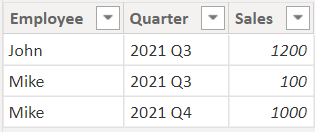Table: Employee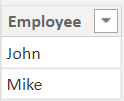There exists a 1 to many relationship from Employee to Sales.

I want to display current vs previous quarter sales by employee in a table visual.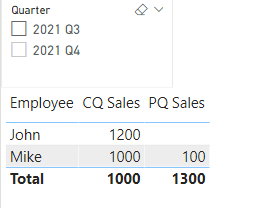When nothing is selected in Quarter slicer, CQ Sales should show sales for 2021 Q4. But it is not working. Measure PQ Sales should display the previous quarter sales for the corresponding employee.

I have written the measures as follows1 ACCEPTED SOLUTIONCommunity Support

Hi @Jos13 ,

To achieve this, you need to create a new seperate calculated table.

``Quarter = DISTINCT('Sales'[Quarter])``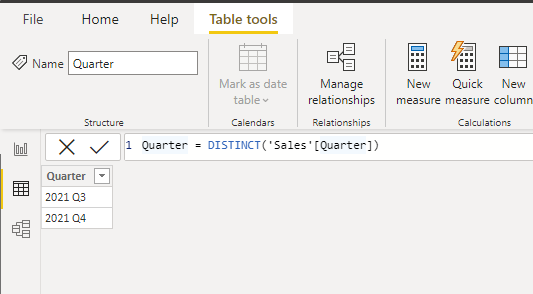The quarter table has no relationship with the main table.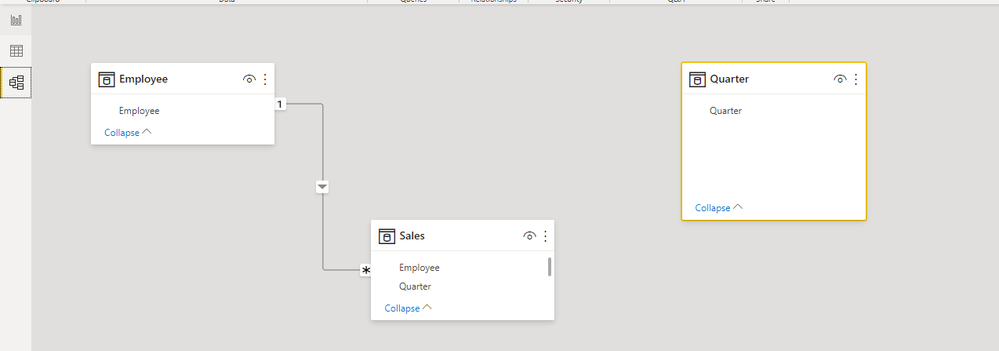In the sales table, I have expanded some data to increase the situation in different years.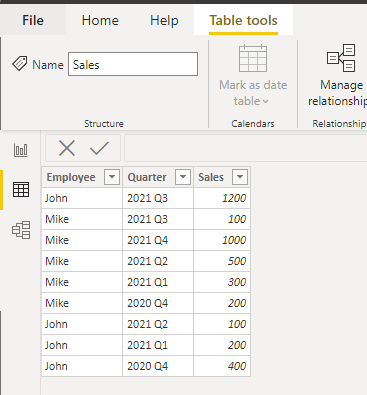Create two measures

``````CQ Sales = var _cq=SELECTEDVALUE('Quarter'[Quarter])
return CALCULATE(SUM('Sales'[Sales]),FILTER('Sales',[Quarter]=_cq))``````
``````PQ Sales = var _cq=SELECTEDVALUE('Quarter'[Quarter])
var _pq=IF(VALUE(RIGHT(_cq,1))=1,VALUE(LEFT(_cq,4))-1&" Q4",LEFT(_cq,4)&" Q"&VALUE(RIGHT(_cq,1))-1)
RETURN CALCULATE(SUM(Sales[Sales]),FILTER('Sales',[Quarter]=_pq))``````

Here's the results.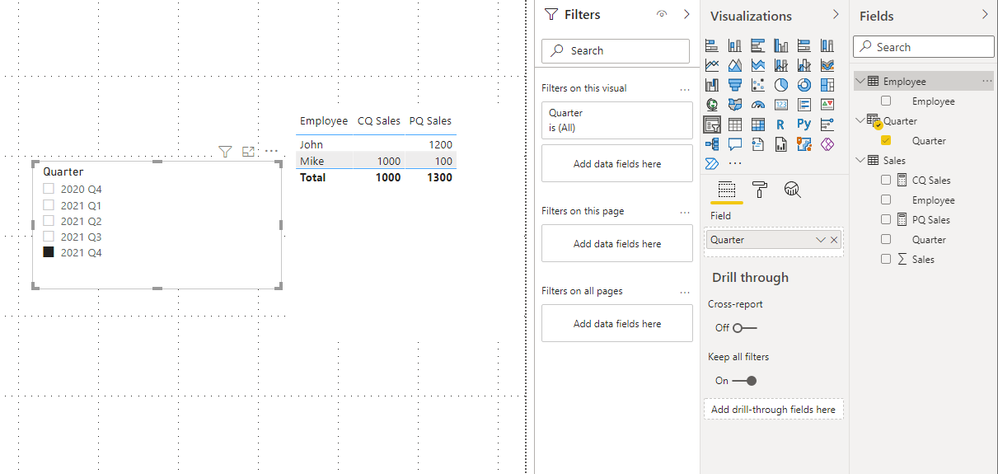Best Regards,

Stephen Tao

If this post helps, then please consider Accept it as the solution to help the other members find it more quickly.

2 REPLIES 2Community Support

Hi @Jos13 ,

To achieve this, you need to create a new seperate calculated table.

``Quarter = DISTINCT('Sales'[Quarter])``The quarter table has no relationship with the main table.In the sales table, I have expanded some data to increase the situation in different years.Create two measures

``````CQ Sales = var _cq=SELECTEDVALUE('Quarter'[Quarter])
return CALCULATE(SUM('Sales'[Sales]),FILTER('Sales',[Quarter]=_cq))``````
``````PQ Sales = var _cq=SELECTEDVALUE('Quarter'[Quarter])
var _pq=IF(VALUE(RIGHT(_cq,1))=1,VALUE(LEFT(_cq,4))-1&" Q4",LEFT(_cq,4)&" Q"&VALUE(RIGHT(_cq,1))-1)
RETURN CALCULATE(SUM(Sales[Sales]),FILTER('Sales',[Quarter]=_pq))``````

Here's the results.Best Regards,

Stephen Tao

If this post helps, then please consider Accept it as the solution to help the other members find it more quickly.Super User

Hi,

You can use QUARTER and PREVIOUSQUARTER functions here.

My test data:Functions:

Current Quarter = CALCULATE(SUM('Quarter calculations'[Value]),QUARTER('Quarter calculations'[Date]))
Previous Quarter = CALCULATE(SUM('Quarter calculations'[Value]),PREVIOUSQUARTER('Calendar'[Date]))

I had a one-to-many relationship  between my 'test data'[date] and 'calendar'[date].
IF you don't have a date column you can construct one from your 'Sales'[Quarter] by using this DAX:

Date from Quarter =
var _quarter = RIGHT('Quarter calculations'[Quarter (different format)],1) return
DATE(LEFT('Quarter calculations'[Quarter (different format)],4),

Switch(_quarter,"1",3,
"2",6,
"3",9,
"4",12),1)

Hopefully htis helps and if it does please accept this as a solution.

Proud to be a Super User!Announcements#### Exclusive opportunity for Women!

Join us for a free, hands-on Microsoft workshop led by women trainers for women where you will learn how to build a Dashboard in a Day!#### Power Platform Conference-Power BI and Fabric Sessions

Join us Oct 1 - 6 in Las Vegas for the Microsoft Power Platform Conference.Top Solution Authors
Top Kudoed Authors
Users online (3,476)